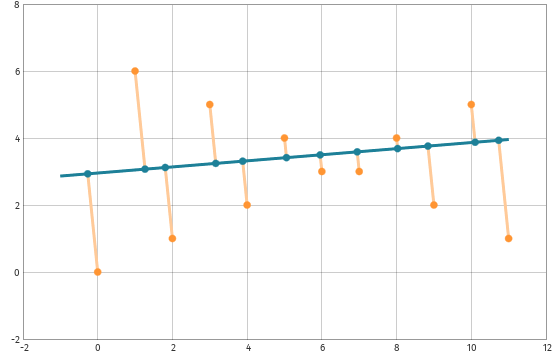# WORHP Kata of May 2023: Points on a sphere ⌚ 2023-05-01

A kata (in the context of programming) is a small training unit to practise coding. In this series of WORHP Katas we present standard problems of optimization which you can use to get familiar with optimization (and our optimization software WORHP).

Kata: We want to distribute 12 points on a unit sphere in $\mathbb{R}^3$. For each pair of points, we compute the reciprocal of their distance. Find the distribution which minimizes the sum of all these reciprocals!

A solution will be provided with the next WORHP Kata.

Solution of last WORHP Kata: For a line $y=mx+t$ and a point $(x_i, y_i)$ we can find the perpendicular foot $(\overline x_i, \overline y_i)$ as
$\begin{array}{rcl} \overline x_i &=& \frac{x_i + m y_i - m t}{m^2+1}\\ \overline y_i &=& m \overline x_i + t \end{array}$
and the Euclidean distance from the point $(x_i, y_i)$ to the line as:
$d_i = \sqrt{(\overline x_i-x_i)^2 + (\overline y_i-y_i)^2}$
To avoid a not differentiable formulation, we introduce an auxiliary optimization variable $d$, which stands for the minimum distance of all points to the line, i.e. $d \ge d_i$ for all points. Hence we have to solve this problem:
$\begin{array}{rl} \displaystyle\min\limits_{m,t,d} &d \\ &d - d_i \ge 0\quad \text{for } i=0,\dots, 11 \end{array}$

Using this CPP code and our solver WORHP, we get after 7 iterations:
$\begin{array}{rcl} m&=& 0.0909091\\ t&=& 2.95455\\ d&=& 2.94241 \end{array}$
In this plot, we see the data points in orange, the optimal line, and the perpendicular feet:When checking the constraints, we see that three points share the same maximal distance from the line, as our solution yields equality $d=d_i$ for $i\in\{0,~1,~11\}$.